Normal view MARC view ISBD view

# Chaotic dynamics: fractals, tilings, and substitutions

Series: Cambridge mathematical textbooks. Publisher: New Delhi Cambridge University Press 2017Description: xiv, 403 p.ISBN: 9781107112674.DDC classification: 515.39 Summary: This undergraduate textbook is a rigorous mathematical introduction to dynamical systems and an accessible guide for students transitioning from calculus to advanced mathematics. It has many student-friendly features, such as graded exercises that range from straightforward to more difficult with hints, and includes concrete applications of real analysis and metric space theory to dynamical problems. Proofs are complete and carefully explained, and there is opportunity to practice manipulating algebraic expressions in an applied context of dynamical problems. After presenting a foundation in one-dimensional dynamical systems, the text introduces students to advanced subjects in the latter chapters, such as topological and symbolic dynamics. It includes two-dimensional dynamics, Sharkovsky's theorem, and the theory of substitutions, and takes special care in covering Newton's method. Mathematica code is available online, so that students can see implementation of many of the dynamical aspects of the text. Requires no prior knowledge of real analysis or metric spaces, making it an ideal transitional text between the calculus sequence and more advanced topics in real analysis and topology Offers an introduction to topological and symbolic dynamical systems that, unlike other available textbooks, is suitable for both senior undergraduates and beginning graduate students Illustrates concrete applications of real analysis and metric space theory to dynamical problems, showing students why advanced mathematics is important and useful Mathematica code is available online, so that students can see implementation of many of the dynamical aspects of the text Read more at http://www.cambridge.org/gb/academic/subjects/mathematics/differential-and-integral-equations-dynamical-systems-and-co/chaotic-dynamics-fractals-tilings-and-substitutions#r7udd6Gw46Hk71CD.99
Tags from this library: No tags from this library for this title.
Item type Current location Collection Call number Status Date due Barcode
Books Vikram Sarabhai Library
General Stacks
Non-fiction 515.39 G6C4 (Browse shelf) Available 197172
##### Browsing Vikram Sarabhai Library Shelves , Shelving location: General Stacks , Collection code: Non-fiction Close shelf browser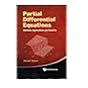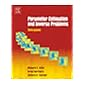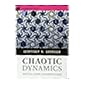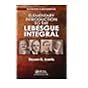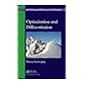515.352 H3O7 Ordinary and partial differential equations (along with CD) 515.353 H2P2 Partial differential equations: methods, applications and theories 515.357 A8P2 Parameter estimation and inverse problems 515.39 G6C4 Chaotic dynamics: fractals, tilings, and substitutions 515.42 T2I6 An introduction to measure theory, Vol 126 515.43 K7E5 Elementary introduction to the Lebesgue integral 515.642 S3O7 Optimization and differentiation

This undergraduate textbook is a rigorous mathematical introduction to dynamical systems and an accessible guide for students transitioning from calculus to advanced mathematics. It has many student-friendly features, such as graded exercises that range from straightforward to more difficult with hints, and includes concrete applications of real analysis and metric space theory to dynamical problems. Proofs are complete and carefully explained, and there is opportunity to practice manipulating algebraic expressions in an applied context of dynamical problems. After presenting a foundation in one-dimensional dynamical systems, the text introduces students to advanced subjects in the latter chapters, such as topological and symbolic dynamics. It includes two-dimensional dynamics, Sharkovsky's theorem, and the theory of substitutions, and takes special care in covering Newton's method. Mathematica code is available online, so that students can see implementation of many of the dynamical aspects of the text.

Requires no prior knowledge of real analysis or metric spaces, making it an ideal transitional text between the calculus sequence and more advanced topics in real analysis and topology
Offers an introduction to topological and symbolic dynamical systems that, unlike other available textbooks, is suitable for both senior undergraduates and beginning graduate students
Illustrates concrete applications of real analysis and metric space theory to dynamical problems, showing students why advanced mathematics is important and useful
Mathematica code is available online, so that students can see implementation of many of the dynamical aspects of the text